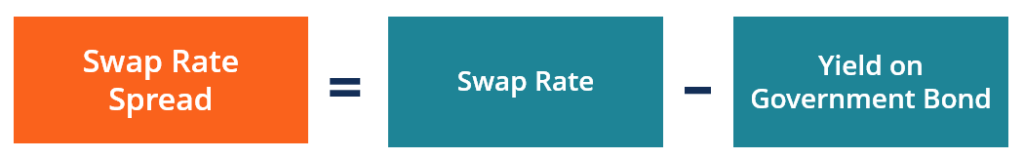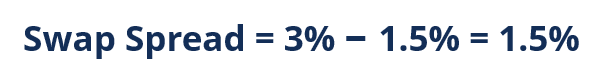The difference between the swap rate and the yield on the government bond with a similar maturity

Swap spread is the difference between the swap rate (the rate of the fixed leg of a swap) and the yield on the government bond with a similar maturity. Since government bonds (e.g., US Treasury securities) are considered risk-free securities, swap spreads typically reflect the risk levels perceived by the parties involved in a swap agreement. Swaps are frequently quoted in the swap spread (another option is the swap rate).### Swap Spread and Market Risk

The swap spreads of interest rate swaps are considered typical indicators of market risk and a measure of the risk aversion prevalent in the market. The swap spreads are commonly used by economists in assessing current market conditions.

Large positive swap spreads generally indicate that the great number of market participants are willing to swap their risk exposures. As the number of counterparties willing to hedge their risk exposures increase, the larger amounts of money the parties are keen to spend to enter swap agreements. Such a trend generally reveals the strong risk aversion among the market participants that can be caused by a high systematic risk in the market.

Additionally, large swap spreads may signify reduced liquidity in the market. It is generally caused by the greater portion of capital employed in the swap deals.

ABC Corp. enters into an interest rate swap agreement with XYZ Corp. It is a 3-year interest rate swap in which ABC Corp. must pay 3% fixed interest rate (i.e., the payer) while XYZ Corp. must pay the floating interest rate equals 1-year LIBOR (i.e., the receiver). The current 3-year yield on the default-free government bond is 1.5%.

In order to calculate the spread of the swap, we need to determine its swap rate. According to the definition, the swap rate is the fixed rate of the swap. Thus, the swap rate of the swap contract between ABC Corp. and XYZ Corp. is 3%, which represents the swap’s fixed rate.

Therefore, the swap spread, which is the difference between the swap rate and the yield on the government’s bond with a similar maturity, must be calculated using the following formula:CFI is the official provider of the Financial Modeling and Valuation Analyst (FMVA)™ certification program, designed to transform anyone into a world-class financial analyst.

To keep learning and developing your knowledge of financial analysis, we highly recommend the additional resources below:

• Sharpe Ratio Calculator